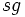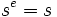# Group action

VIEW RELATED: Analogues of this | Variations of this | Opposites of this |[SHOW MORE]

We follow the convention of action on the left. Please refer Convention:Group action on left

## Terminology

The term group action or action of a group is used for the notion defined here. An alternative term sometimes used is permutation representation.

## Definition (left action)

### Definition in terms of action

A group action on the left (simply called a group action if the convention is of left actions)$\alpha$ of a group$G$ on a set$S$ is a map$\alpha: G \times S \to S$ such that the following two conditions are satisfied:

•$\alpha(gh,s) = \alpha(g,\alpha(h,s))$
•$\alpha(e,s) = s$ (here,$e$ is the identity element of$G$).

### Definition in terms of homomorphisms

A group action on a set or an action of a group on a set is a group homomorphism from the group to the symmetric group on the set.

In symbols, a group action of a group$G$ on a set$S$ is a homomorphism$\rho: G \to \operatorname{Sym}(S)$ where$\operatorname{Sym}(S)$ denotes the symmetric group on$S$.

### Equivalence of definitions

Further information: Equivalence of definitions of group action

### Convenience of notation

For convenience, we omit the symbols$\alpha$ or$\rho$, and write the action of$g$ on$s$ as$g.s$, or sometimes just as$gs$.

We can then rewrite the first condition as:$g(hs) = (gh)s$

This is just like associativity, and hence we can drop the parenthesization, so we often write$ghs$ for either of the above.

## Definition (right action)

### Definition in terms of action

A group action on the right (simply called a group action if the convention is of right actions) of a group$G$ on a set$S$ is a map$\alpha:S \times G \to S$ satisfying the following:

•$\alpha(\alpha(s,g),h) = \alpha(s,gh)$ for all$s \in S, g,h \in G$.
•$\alpha(s,e) = s$ for all$s \in S$.

### Definition in terms of homomorphisms

A group action on a set or an action of a group on a set is a group homomorphism from the group to the symmetric group on the set.

In symbols, a group action of a group$G$ on a set$S$ is a homomorphism$\rho: G \to \operatorname{Sym}(S)$ where$\operatorname{Sym}(S)$ denotes the symmetric group on$S$.

(Note that this definition remains the same as the previous one, because if the convention is of right actions, the multiplication table of the symmetric group also has the order of composition reversed).

### Convenience of notation

Group actions on the right are usually denoted either by juxtaposition or by exponentation. The juxtaposition notation writes$\alpha(s,g)$ as$sg$. The exponentiation notation writes$\alpha(s,g)$ as$s^g$. The latter is more common for right actions, as it helps maintain the separation between the set and the group. In terms of this notation, the axioms read:

•$(s^g)^h = s^{gh}$.
•$s^e = s$.

## Related notions

• Monoid action: This is the corresponding notion of action for a group without inverses

Also refer Category:Group action properties

## Terminology

### Orbit

Further information: orbit under group action

Suppose$G$ is a group acting on a set$S$. Then, for any point$s \in S$, the orbit of$s$ under the action of$G$, denoted$G.s$, is defined as:$\{ t \in S \mid \ \exists \ g \in G, g.t = s \}$

In other words, the orbit of a point is the set of all points that can be reached from that point under the action of the group.

Because of the reversibility of the action of elements of the group, it turns out that if$t$ is in the orbit of$s$,$s$ is also in the orbit of$t$. Specifically, if$g.s = t$, then$g^{-1}.t = s$. Hence we can talk of the relation of being in the same orbit. This relation is reflexive (because of the identity element), symmetric (because of invertibility) and transitive (because of the homomorphism nature of the group action), and hence gives an equivalence relation. The equivalence relation thus partitions$S$ into a disjoint union of orbits.

### Transitive group action

Further information: Transitive group action

A group action is termed transitive if it has exactly one orbit (typically, we exclude the action of groups on the empty set when talking of transitive group actions, though, technically, the action on the empty set is also transitive). In other words, the action of a group$G$ on a set$S$ is termed transitive if for any$s,t \in S$, there exists$g \in G$ such that$g.s = t$.

### Faithful group action

Further information: Faithful group action

A group action is termed faithful if no non-identity element of the group fixes everything. In other words, the action of a group$G$ on a set$S$ is termed faithful if for every non-identity element$g \in G$, there exists$s \in S$ such that$g.s \ne s$.

### Stabilizer

Further information: Point-stabilizer

Given a group$G$ acting on a set$S$, the point-stabilizer of$s \in S$, also termed the isotropy group or isotropy subgroup at$s$, denoted$Stab_G(s)$, is defined as:$\{ h \in G \mid \ hs = s \}$

In other words, it is those elements of the group that fix$s$.

Some true facts:

• The orbit of$s$ can be naturally identified with the coset space of$G/H$, where$H$ is the isotropy subgroup of$s$. For full proof, refer: Fundamental theorem of group actions
• If$gs = t$, the isotropy subgroup at$s$ and$t$ are conjugate by$g$; in other words:$g Stab_G(s) g^{-1} = Stab_G(t)$

## Examples

### Symmetric group action

Suppose$S$ is a set and$G = \operatorname{Sym}(S)$. Then,$G$ acts on$S$ by definition: given$g \in G$ and$s \in S$, we define$g.s = g(s)$, i.e., the image of$s$ under the permutation$g$.

### Left-regular group action

Suppose$G$ is a group. Then,$G$ acts on itself by left multiplication. Here, the action is defined by:$g . h = gh$